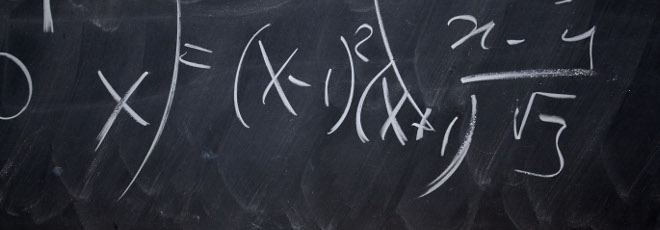## “Potential Theory and Boundary Element Method for the Laplace equation. An introduction”

Wednesday 20 November 2019, h. 14:30 - Room 2BC30 - Andrei-Florin Albisoru (Babes-Bolyai University, Cluj, Romania)

Abstract

We aim to give an overview of Potential theory for Laplace’s equation. We introduce the fundamental solution of this equation. Next, we define the layer potentials and we state their properties. Using the layer potentials we will construct a solution of the interior Dirichlet problem for the Laplacian. We also describe a numerical method of solving Laplace’s equation, namely the Boundary Element Method. Finally, we present some numerical results.